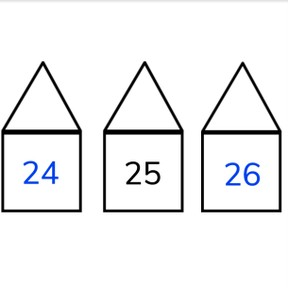Neighbor numbers to 50

# Neighbor numbers to 50

Neighbor numbers to 50

No account needed.8,000 schools use Gynzy92,000 teachers use Gynzy1,600,000 students use Gynzy

## General

Students learn what neighbor numbers are and can determine neighbor numbers from 20-49.

K.CC.A.1
K.CC.A.2

## Relevance

Discuss with students that it is important to know neighbor numbers to so you know which number comes in order. You can use this to know when someone is next in line when you draw numbers.

## Introduction

Count forwards and backwards to 50 with the students. Start with a random number and count forwards or backwards. Do this a few times in different ways. Ask students to count and sing, to whisper, to whisper, or to count by turns. Hand out number cards to 20. Write a number from 1-19 on the interactive whiteboard and ask the students who have the neighbor numbers of the given number to hold up their cards. Practice a few times with different numbers.

## Development

Explain that neighbor numbers are numbers that are one back and one more than a given number. Use the houses to help explain the idea of neighbors. Explain how you can determine neighbor numbers by counting one back or by counting one more. Ask students to name 3 neighbor numbers and write them on the interactive whiteboard.

Check that students understand neighbor numbers to 50 by asking the following questions:
- What is a neighbor number?
- How do you determine a neighbor number?
- Do all numbers have a neighbor number?

## Guided Practice

Students first practice filling in the number that comes before a given number, then practice giving the number that comes after. They are then asked to fill in both neighbor numbers.

## Closing

Check that students understand neighbor numbers and can explain what they mean. Tel students that neighbor numbers are the numbers before and after a given number on the number line. Emphasize that it is useful to know neighbor numbers so we know which one came before, and which one comes next. Check that students can find the neighbor numbers by counting one back and one more of a given number. Have students select the neighbor numbers and drag them to the correct houses on the interactive whiteboard.

## Teaching Tip

Students who have difficulty with neighbor numbers likely are not comfortable counting to 50. Have the students practice counting forwards and backwards to 50 and they can also be supported by using the number line. Students who are looking for challenge can be encouraged to try finding neighbor numbers with numbers to 100.

## Instruction materials

Number cards to 20.

### The online teaching platform for interactive whiteboards and displays in schools

• Save time building lessons

• Manage the classroom more efficiently

• Increase student engagement